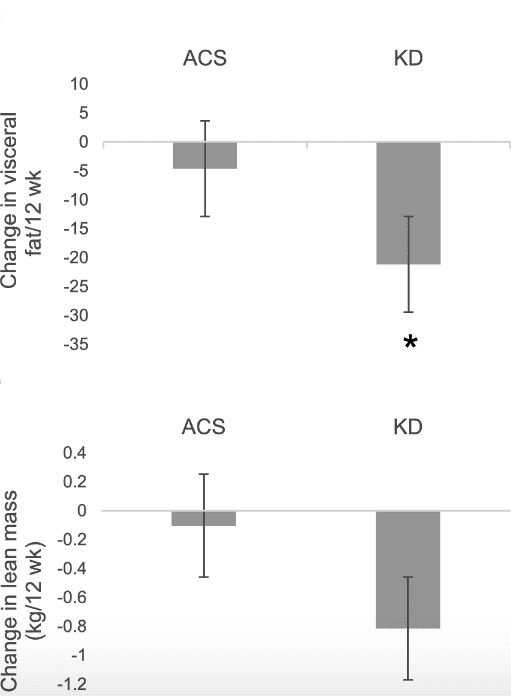# Misuse of Standard Error in Clinical Trials

Reporting effect sizes with their accompanying standard errors is necessary because it lets the reader interpret the magnitude of the treatment effect and the amount of uncertainty in that estimate. It is magnitudes better than not providing any effect size at all and only focusing on statements of statistical significance.

Although many authors provide standard errors with the intention of relaying the amount of uncertainty in the estimate, there are several misconceptions about when the standard error should be reported, and so it is often misused.

I believe it is worth explaining what exactly standard error is and how it differentiates from something like standard deviation. Standard deviation is a descriptive statistic that tells us about the variability of the data. Let’s quickly take a look at how it’s calculated using random data.

# Calculating Standard Deviation

$4, 5, 10, 6, 7, 10, 10$

$4 + 5 + 10 + 6 + 7 + 10 + 10 = 52$

The mean is: $$52 / 7 = 7.43$$

Next, we calculate the sum of squared errors, which can be thought of as the total deviance from the mean. We calculate this by taking each value in the data ($$4, 5, 10, 6, 7, 10, 10$$) and subtracting the mean from each value, which gives us the deviance.

$(4 - 7.43) = -3.43$

$(5 - 7.43) = -2.43$

$(10 - 7.43) = 2.57$

$(6 - 7.43) = -1.43$

$( 7 - 7.43) = -0.43$

$(10 - 7.43) = 2.57$

$(10 - 7.43) = 2.57$

All of the above values are deviances. We square each of them and add them together to get the sum of squared errors.

$(-3.43)^2 + (-2.43)^2 + (2.57)^2 + (-1.43)^2 + (-0.43)^2 + (2.57)^2 + (2.57)^2 =$

Sum of Squared Errors = $$39.68$$

We get the variance by taking the sum of squared errors and dividing by the number of data points $$-1$$.

$39.68 / 7-1 = 6.61$

Variance = $$6.61$$

Finally, we get the standard deviation by taking the square root of the variance, $(\sqrt{6.61})$

Standard deviation = $$2.57$$

There we have it. Again, the standard deviation is a descriptive statistic which tells us about the variability of the data.

# Calculating Standard Error

The standard error, on the other hand, is the standard deviation of the sampling distribution. It can be thought of as the standard deviation of several means. It is an analytic statistic that tells us about the uncertainty in our point estimate. It is calculated by taking the standard deviation and dividing it by the square root of the total number of data points.

$2.57 / (\sqrt7) = 0.97$

Standard error of the mean = $$0.97$$

This and the mean would typically be reported as such $$7.43 ± 0.97$$

# Misuse of Standard Error

In clinical trials, participants are often recruited nonrandomly but are assigned to groups via random assignment. This random allocation allows us to make inferences about those treatment differences (Kempthorne, 1977; Senn & Auclair, 1990). So if the difference between the intervention group and the control group is 7.43 generic units, then we are justified in reporting the standard error (an analytic statistic) as 7.43 ± 0.97.

However, let’s say in a similar scenario (randomized controlled trial) that the intervention group after 12 weeks has an average of 7.43 generic units. Not a difference between the intervention group and the control group, but rather, an average of 7.43 generic units for the intervention group itself. The control group has an average of yy generic units. If we reported the average of the intervention group as 7.43 ± 0.97 (same numbers we calculated above), this would be incorrect.

It is appropriate to report an analytic statistic when there is some form of randomization involved to justify it. In clinical trials, where the focus is on the treatment differences, it would be appropriate to report the standard error for the differences but not the group averages themselves because there is no random sampling involved. Thus, we cannot make inferences from those group averages.

“For inferences to be drawn about averages (rather than differences) it is necessary to make the strong assumption of random sampling; this requires survey validity. In short, the analysis of clinical trials should estimate treatment differences.” (Senn & Auclair, 1990)

In this scenario, it would make sense to report the group average with a descriptive statistic such as the standard deviation: 7.43 (2.57).

We are allowed to make inferences about the group averages, and thus report it as 7.43 ± 0.97 if there was random sampling involved, which is often done in surveys etc., but not very often in clinical trials.

Is this an actual problem? Yes. Although several papers have misreported these statistics, I’ll focus on a recent study (Cohen et al., 2018) that compared the ketogenic diet to the American Cancer Society diet in women with ovarian or endometrial cancer. Some of the outcomes were changes in total fat, android fat, visceral mass, and lean mass.Here, they graph the average change in lean mass and visceral mass in each of the groups and add the standard error of the mean as error bars, “Values are means ± SEMs.” Again, it would’ve been appropriate here to report the standard deviation rather than the standard error of the mean. That would’ve shown the variability of the group data.

Help support the website!